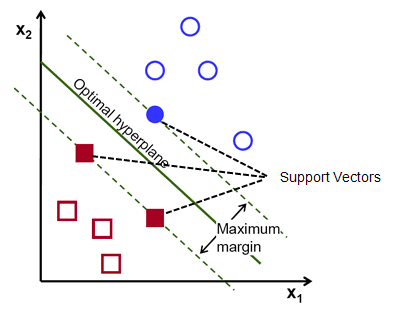#This tutorial describes theory and practical application of Support Vector Machines (SVM) with R code. It's a popular supervised learning algorithm (i.e. classify or predict target variable). It works both for classification and regression problems. It's one of the sought-after machine learning algorithm that is widely used in data science competitions.What is Support Vector Machine?

The main idea of support vector machine is to find the optimal hyperplane (line in 2D, plane in 3D and hyperplane in more than 3 dimensions) which maximizes the margin between two classes. In this case, two classes are red and blue balls. In layman's term, it is finding the optimal separating boundary to separate two classes (events and non-events).Support Vectors are observations that supports hyperplane on either sides. In the image above, filled red and blue boxes and circles are support vectors.

Why Hyperplane?

Hyperplane is just a line in 2D and plane in 3D. In higher dimensions (more than 3D), it's called hyperplane. SVM help us to find a hyperplane (or separating boundary) that can separate two classes (red and blue dots).

What is Margin?

It is the distance between the hyperplane and the closest data point. If we double it, it would be equal to the margin.

Objective : Maximize the margin between two categories

How to find the optimal hyperplane?

In your dataset, select two hyperplanes which separate the data with no points between them and maximize the distance between these two hyperplanes. The distance here is 'margin'.

How to treat Non-linear Separable Data?
Imagine a case - if there is no straight line (or hyperplane) which can separate two classes? In the image shown below, there is a circle in 2D with red and blue data points all over it such that adjacent data points are of different colors.
SVM handles the above case by using a kernel function to handle non-linear separable data. It is explained in the next section.

Views: 12772

Comment

Join Data Science Central